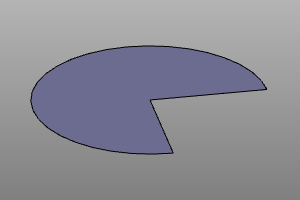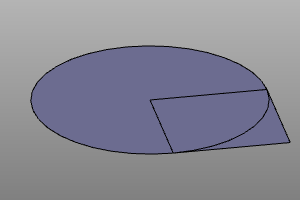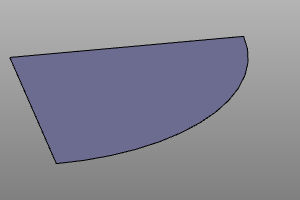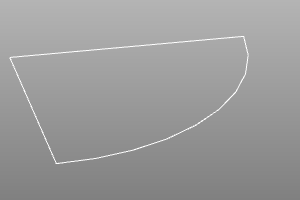# Boolean operations.

CSG geometry is operated on boolean operations. Zencad provides operations for joining, subtracting and intersecting 3d and 2d objects. There are two groups of these operations in zencad:

• over arrays of bodies using the functions union, difference, intersect
• over pairs of bodies using the operators _ + _ - _ ^ _

! Note: ! Do not attempt to boolean a compound line from simple lines or sew a shell from faces. For these manipulations, there are special stitching procedures outlined in the relevant sections.

## Union.

Signature:

``````# Функция:
result = union(array)

# Оператор:
result = shp0 + shp1
``````

Example:

``````#with operators:
sphere(r=10) + cylinder(r=5, h=10, center=True) + cylinder(r=5, h=10, center=True).rotateX(deg(90))

#with function:
union([
sphere(r=10),
cylinder(r=5, h=10, center=True),
cylinder(r=5, h=10, center=True).rotateX(deg(90))
])
``````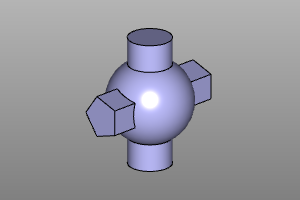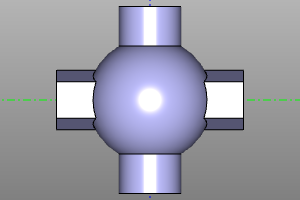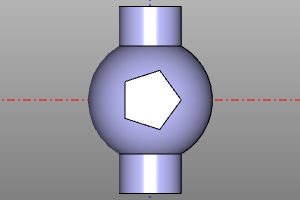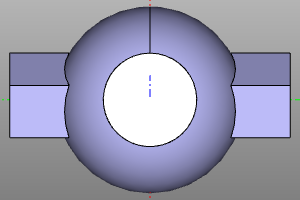## Difference.

Signature:

``````# Функция:
result = difference(array)

# Оператор:
result = shp0 - shp1
``````

Example:

``````#with operators:
sphere(r=10) - cylinder(r=5, h=10, center=True) - cylinder(r=5, h=10, center=True).rotateX(deg(90))

#with function:
difference([
sphere(r=10),
cylinder(r=5, h=10, center=True),
cylinder(r=5, h=10, center=True).rotateX(deg(90))
])
``````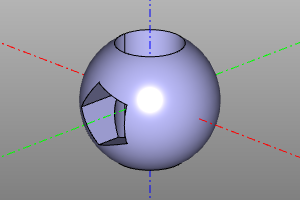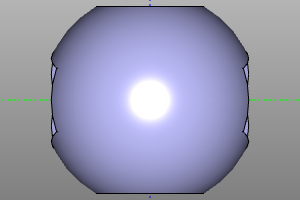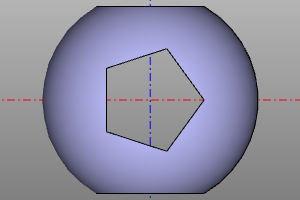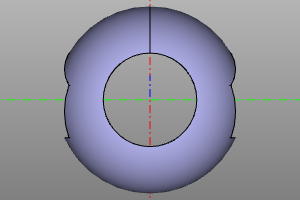## Intersect.

Signature:

``````# Функция:
result = intersect(array)

# Оператор:
result = shp0 ^ shp1
``````

Example:

``````#with operators:
sphere(r=10) ^ cylinder(r=5, h=10, center=True) ^ cylinder(r=5, h=10, center=True).rotateX(deg(90))

#with function:
intersect([
sphere(r=10),
cylinder(r=5, h=10, center=True),
cylinder(r=5, h=10, center=True).rotateX(deg(90))
])
``````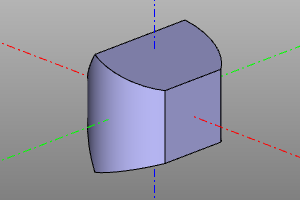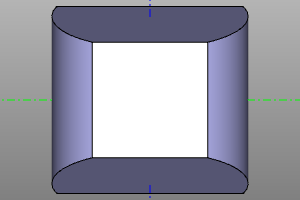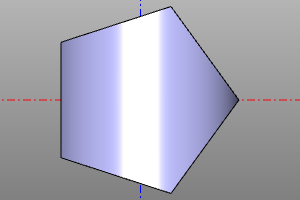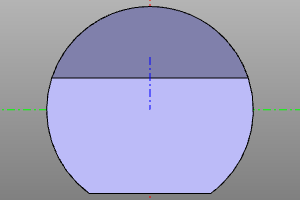## Crossing shells.

Let's twin the operation intersect, which calculates the intersection of the shells of bodies.

Signature:

``````# Функция:
result = section(a, b)
``````

Example:

``````m0 = section(box(10, center=True) - sphere(4))
m1 = section(box(10, center=True), sphere(7))
``````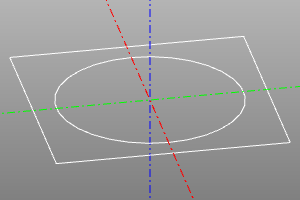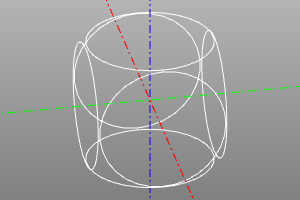## Boolean operations on 2D solids.

Just like with 3D objects, the above operations can be applied to 2D objects as long as they are in the same plane.

Example:

``````m0 = sphere(10) - square(10)
m1 = sphere(10) + square(10)
m2 = sphere(10) ^ square(10)
m2 = section(sphere(10), square(10))
``````